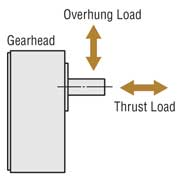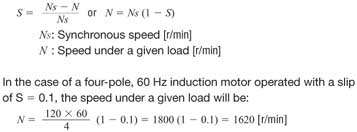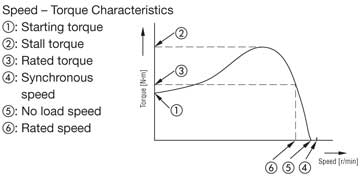Free Shipping for Online Orders. Terms and conditions apply.

# AC Motor Glossary of Terms

Gear Ratio
The gear ratio is the ratio by which the gearhead reduces the motor speed. The speed at the gearhead's output shaft is 1 Gear Ratio times the motor speed.

Maximum Permissible Torque
This is the maximum load torque that can be applied to the gearhead. It is dependent upon such mechanical strength factors as the materials of gearheads and bearings, and size. Therefore, it varies according to the gearhead type and gear ratio.

Service Factor
This is a coefficient used to estimate the gearhead life. These values are determined in accordance with the results of life tests under various loads and conditions of use.

Transmission Efficiency
This is the efficiency when the torque is transmitted with the gearhead combined. It is expressed as a percentage (%) and is determined by the friction in the gears and bearings used in the gearhead and the resistance of the lubrication grease.  Transmission efficiency is, when using a GN gearhead, usually 90% for one stage of reduction gears, and is 81% for two stage gearheads. As the gear ratio increases, the number of reduction gear stages increases, with a consequent reduction in the gear efficiency to 73% and 66% for each gear stage added.

This is a load on the gearhead output shaft in the vertical direction. The maximum overhung load on a gearhead shaft is called the permissible overhung load, and it varies with the gearhead type and distance from the shaft end. This is equivalent to tension under belt drive.

This is the load that is placed in the direction of the gearhead output shaft. The maximum thrust load on the gearhead is called the permissible thrust load, which varies with the gearhead type.## Output Power

Output Power
The amount of work that can be performed in a given period of time is determined by the motor's speed and torque. Each motor specification indicates the value of rated output power. Output power is expressed in watts or in horsepower.

Output Power [Watts] = 1.047 × 10-1 × T × N
1 HP = 746 Watts
where: 1.047 × 10-1 : Constant
T [N·m] : Torque
N [r/min] : Speed

Rated Output Power
This term refers to output power generated continuously when the optimal characteristics are achieved at the rated voltage and frequency in continuous operation. The speed and torque that produce the rated output power are called the rated speed and rated torque. Generally, the term "output power" refers to rated output power.

## Overrun

Overrun
This is the number of excess rotations the motor makes from the instant the power is cut off to the time that it actually stops. It is normally indicated either by angle or by rotations.

## Ratings

Ratings
Motor rating represents the operation limit certified on the motor regarding dynamic characteristics such as temperature, mechanical strength, vibration and efficiency. There are two categories: continuous rating and limited duty rating. Operation limit on output power, as well as voltage, frequency and speed are established. These are known as rated output powerrated voltagerated frequency and rated speed, respectively.

Continuous and Limited Duty Ratings
The time during which output can continue without abnormality is called a time rating. When continuous operation at rated output is possible, it is known as a continuous rating. When operation at rated output is possible only for a limited time, it is known as the limited duty rating.

## Speed

Synchronous Speed
This is an intrinsic factor determined by line frequency and the number of poles.
It is indicated as the speed per minute.

Ns = 120f   [r/min]
P

NS : Synchronous speed [r/min]
f : Frequency [Hz]
P : Number of poles
120 : Constant

For example, for a four-pole motor with a line frequency of 60 Hz, the synchronous speed will be:

Ns =   120 × 60     =  1800  [r/min]
4

See (4) in the permissible torque chart.

This is the speed under no load conditions. The speed of induction or reversible motors under no load conditions is lower than synchronous speed by a few percent (approximately 20 to 60 r/min). See (5) in the permissible torque chart.

Rated Speed
This is the appropriate speed of the motor at rated output power. From the standpoint of utility, it is the most desirable speed. See (6) in the permissible torque chart.

Slip
The following formula is one method of expressing speed:## Torque

Starting Torque
This is the torque generated instantly when the motor starts. If the motor is subjected to a friction load greater than this torque, it will not operate. See (1) in the figure below.

Stall Torque
This is the maximum torque under which the motor will operate at a given voltage and frequency. If a load greater than this torque is applied to the motor, it will stall. See (2) in the figure below.

Rated Torque
This is the torque generated when the motor is continuously producing rated output power at the rated voltage and frequency. It is the torque at rated speed. See (3) in the figure below.

Static Friction Torque
Static friction torque is the torque output required to hold a load when the motor is stopped by an electromagnetic brake or similar device.

Permissible Torque
The permissible torque is the maximum torque that can be used when the motor is running. It is limited by the motor's rated torque, temperature rise and the strength of the gearhead combined with the motor.## Other Terms

CW, CCW
These show the direction of motor rotation. CW is clockwise as seen from the output shaft, while CCW is counterclockwise.